Homework Help Question & Answers

2. (14 points) Consider the following weighted graph G: 구 12 2 12 나 2 12 Use Prim's algorithm t...

Discrete math! Please ensure the answers are correct and thorough/justified! Thank you.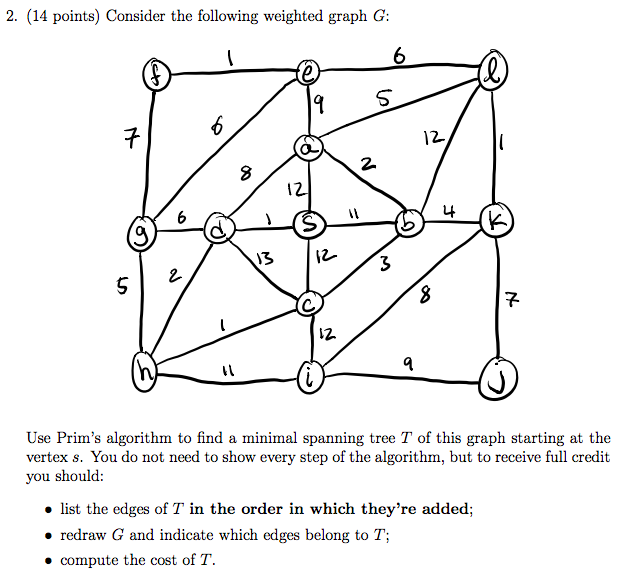2. (14 points) Consider the following weighted graph G: 구 12 2 12 나 2 12 Use Prim's algorithm to find a minimal spanning tree T of this graph starting at the vertex s. You do not need to show every step of the algorithm, but to receive full credit you should: o list the edges of T in the order in which they're added; redraw G and indicate which edges belong to T; * compute the cost of T.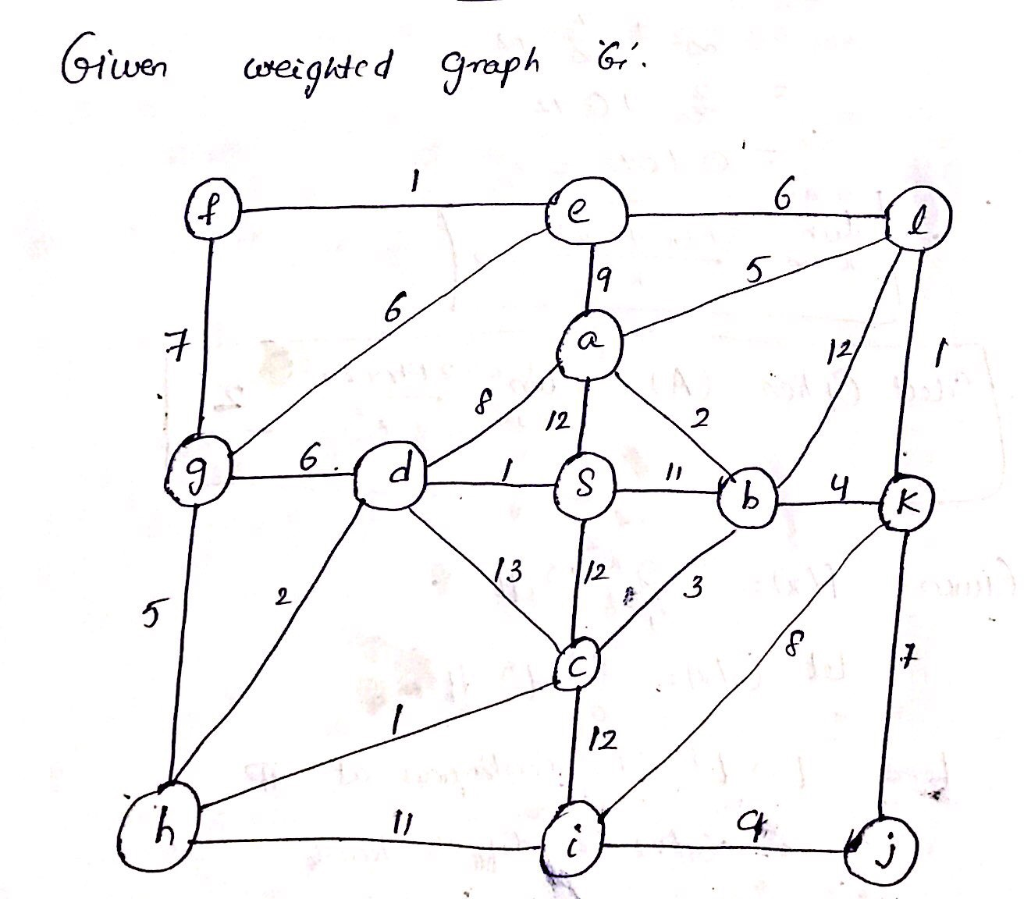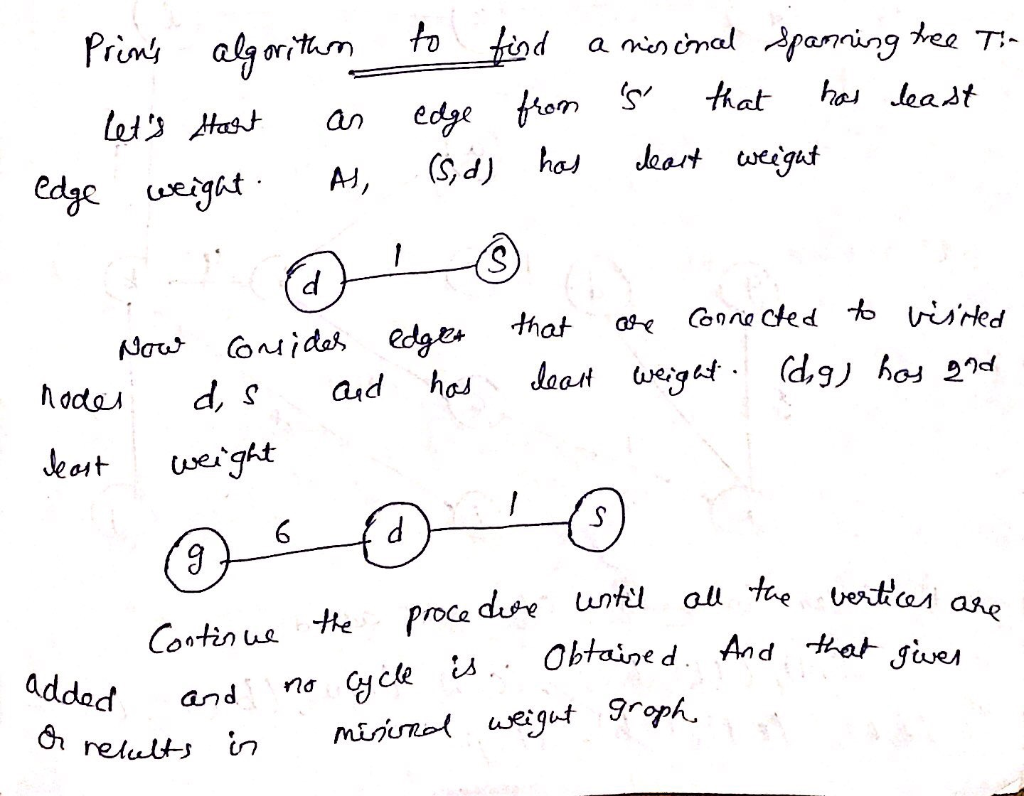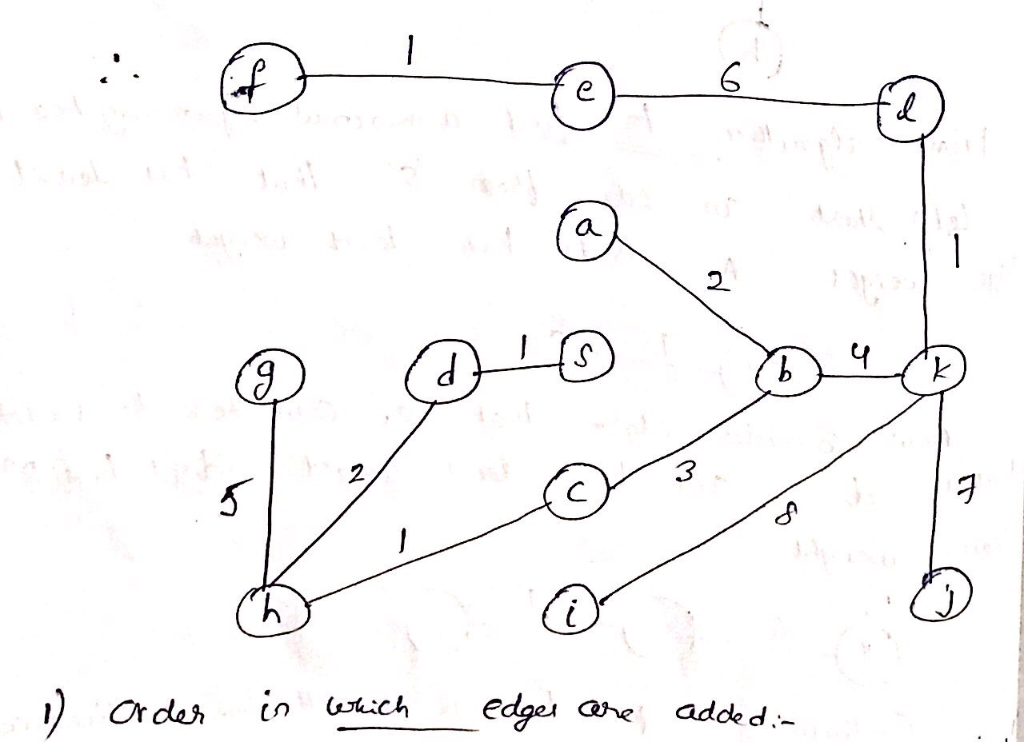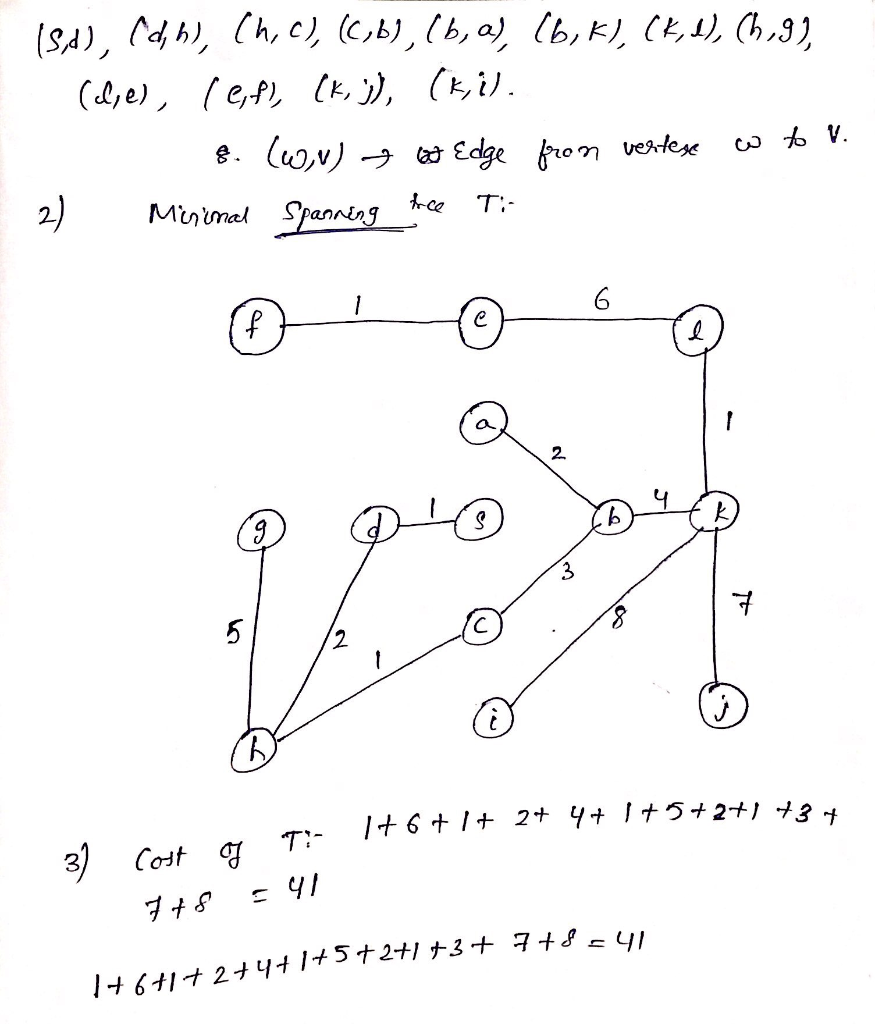Add Answer of: 2. (14 points) Consider the following weighted graph G: 구 12 2 12 나 2 12 Use Prim's algorithm t...
More Homework Help Questions Additional questions in this topic.

• This is a weighted graph algorithm starting from NBA to NFL. Using "Dijkstra’s algorithm" find me...

Need Online Homework Help?

Get FREE EXPERT Answers
WITHIN MINUTES
Related Questions